Synchronous Decision Diagrams: a Data Structure for Representing Finite Sequential Digital Functions

Jean Vuillemin

DMI, École normale supérieure

Algorithms Seminar

May 22, 2000

[summary by Philippe Dumas and Philippe Flajolet]

A properly typeset version of this document is available in postscript and in pdf.

If some fonts do not look right on your screen, this might be fixed by configuring your browser (see the documentation here).

Abstract
Binary Diagrams (BDD's) are an important way to represent boolean functions, that is, combinational circuits. Vuillemin proposes Synchronous Decision Diagrams (SDD's) that are capable of representing all causal circuits with finite memory. The framework provides a general basis for the analysis and synthesis of digital circuits. On the mathematical side, it provides unexpected connections between hardware design and the classical notion of automatic sequences in number theory.

Researchers working in circuit theory are concerned with design (given a function, how can it be realized efficiently?) and analysis (what is the function computed by a given circuit?). This talk presents a mathematical framework for the design and analysis of boolean circuits, either combinational (i.e., without memory) or sequential (i.e., with memory). It is superbly elegant as well as conceptually simple. We shall start here with a review of Binary Decision Diagrams (BDD's) that constitute a canonical way to represent boolean functions and serve the purpose of a gentle introduction to the subject. Then, we shall proceed with Synchronous Decision Diagrams (SDD's) that can represent any type of circuit likely to be encountered in practice (i.e., circuits with finite memory of the past whose output does not depend on the future). Due to severe time constraints imposed by the editor of the seminar proceedings,1 the authors of this summary regret that they cannot do full justice to the work presented and refer to the paper  for an introduction to the main ideas.

## 1   Binary Decision Diagrams

Let B be the boolean domain B={0,1}. A boolean function of n variables is a function from  Bn into  B. Such a function may be specified by its truth table that is the sequence of its values on its 2n possible inputs. Let Fn be the set of n-ary functions and fn the corresponding cardinality. Clearly, one has fn=22n, hence the identity
fn+1= 2
 2n+1
º æ
è
2
 2n
ö
ø
 2
= ( fn )
 2
.
This trivial identity suggests the existence of a fundamental isomorphism
Fn+1 ~ Fn×Fn.
Indeed any boolean function f(x1,...,xn,xn+1) with fÎFn+1 can be specified by a pair (f0,f1), where f0ÎFn and f1ÎFn are ``specializations'' of f,
f0(x1,...,xn):=f(x1,...,xn,0),      f1(x1,...,xn):=f(x1,...,xn,1).
Consequently, when the decomposition is iterated, any boolean function of n variables becomes representable as a perfect binary tree, the binary decision tree bdt(f), whose height is n, whose internal nodes correspond to partial specializations of f, and whose external nodes are either the constant function 0 or the constant function 1. Observe that reading the B labels of the extrenal nodes of bdt(f) from left to right produces precisely the truth table of f.

The binary decision diagram2 of f, bdd(f), is then nothing but the directed acyclic graph (dag) representation of this tree obtained in the usual way by sharing repeated subtrees and representing them only once. It is classically known that such a dag representation of a tree of size N can always be constructed in time O(N); see for instance  for a discussion. Here, one has N=2n for functions in Fn, so that the sharing algorithm approach is of exponential time complexity when f is given by its truth table or, equivalently, by its tree bdt(f). In many cases, fortunately, one can operate with polynomial time complexity.

Here is an example. Consider the adder function on three variables,
f(a,b,c)=aÅ b Å c.
We purposely refrain from operating with the truth-table description of f in order to emphasize that BDD's are directly accessible via a symbolic calculus on boolean functions. Here, two ``subfunctions'' are first obtained upon setting the variable c to either 0 or 1:
f0(a,b)=aÅ b,     f1(a,b)=aÅ bÅ 1.
Next, specialize b, which yields here only two (and not four!) distinct functions, namely,
f00(a)=a,     f10(a)=aÅ1     (with f01º f10 and f11º f00).
Finally, specialize a, which eventually leads to a reduction to the two constant functions
f000()=0,     f010()=1    (with f100º f010 and f110º f000).Figure 1: The adder function, f(a,b,c)=aÅ bÅ c: its Binary Decision Tree (left) and its Binary Decision Diagram (right).

This example shows, more generally, that the BDD of the n-fold adder f(x1,...,xn) can be determined in time linear in n via basic boolean algebra alone, this despite the fact that the truth table has size 2n. The construction in the case of the function f(a,b,c)=aÅ b Å c is described in Figure 1.

Bryant has invented the BDD concept in 1986 (see [1, 2]). The BDD of an n-ary function can often be computed in time much less than O(2n) (cf. the adder example), since it captures the regularities that are likely to be present in most functions occurring in practice.3 Also, given the BDD's of f and g it is possible, in low polynomial time, to determine BDD's for various compositions of f and g like fÅ g, f ° g, etc. Finally, once an ordering on variables has been fixed,4 the BDD becomes a canonical representation of the function it represents, so that equivalence of boolean functions becomes decidable in time linear in the sizes of the compact BDD representations. In particular, this observation makes it possible to compare any combinational circuit design against a canonical specification (the ``semantics'' of the function) in a computationally efficient manner. This constitutes one of the powerful implications of the BDD concept.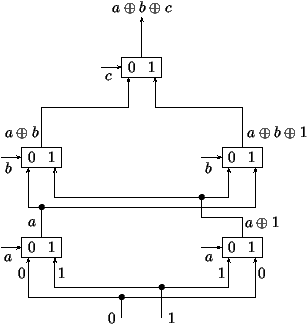Figure 2: A realization of the adder function based on the BDD representation and multiplexers.

Finally, we mention that once the BDD form of a boolean function has been obtained, a circuit realization of proportional size is immediate: all that is needed is ``Shannon's switch'' also known as ``multiplexer,''
mux (x,f,g):=`if x then f else g' =(x Ù f) Ú (x Ù g),
together with entries grounded at 0 and 1. A diagrammatic representation is as follows:The way the BDD of the adder function ``compiles'' into a circuit based on multiplexers is displayed in Figure 2.

## 2   Polynomial Representations of BDD's

As a preparation for the treatment of synchronous decision diagrams, we now introduce a representation of boolean functions by means of univariate polynomials with coefficients in the binary field F2. Let f be a boolean function in n variables. Its truth-table polynomial F=Tf is defined as follows: interpret each n-tuple (x1,x2,...,xm) of boolean values as the binary representation of an integer,
b(x1,...,xn):=(x1x2... xn)2 = x12n-1+...+xn,
(observe the convention that lower order bits are on the right), and set
T f(z)=
 å x1,...,xnÎ B
f(x1,x2,...,xn) z
 b(x1,...,xn)
.
For instance the adder function f(a,b,c)=aÅ bÅ c has the standard truth table
 x1x2x3 000 001 010 011 100 101 110 111 b(x1,x2,x3) 0 1 2 3 4 5 6 7 f(x1,x2,x3) 0 1 1 0 1 0 0 1
so that its truth-table polynomial is
Tf(z)=z+z2+z4+z7.

The BDD algorithm is amenable to interpretation in this formalism. Define the two ``sectioning'' operators on polynomials F2[z] by
S0 æ
ç
ç
è
 å k
fkzk ö
÷
÷
ø
=
 å k
f2kzk,     S1 æ
ç
ç
è
 å k
fkzk ö
÷
÷
ø
=
 å k
f2k+1zk.
(The definition is also valid for power series of F2[[z]], a fact to be used later.) The specialization of the last bit in a function f(x1,...,xn) is then seen to be isomorphic to sectioning. Indeed, a simple calculation shows that
 S0 T ( f(x1,...,xn-1,xn) )
 = T ( f(x1,...,xn-1,0) )
 S1 T ( f(x1,...,xn-1,xn) )
 = T ( f(x1,...,xn-1,1) ) .
Consequently, the BDD construction can be regarded as being equivalent to decomposing a polynomial by means of S0S1 until an eventual reduction to the constants 0 and 1 is attained. In this framework, the BDD algorithm applied to the adder example corresponds to the tree and the diagram of Figure 3.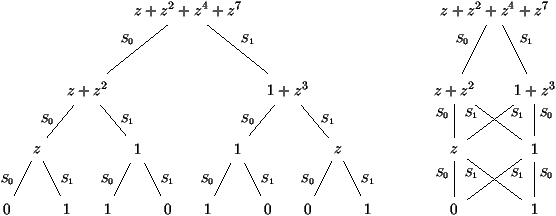Figure 3: Polynomial representations of the Binary Decision Tree and the Binary Decision Diagram of the ternary adder.

## 3   Synchronous Decision Diagrams

In all generality, a sequential function maps infinite sequences of binary inputs into infinite sequences of binary outputs. It thus takes as input a ``stream'' of bits (xt)t³0 and produces another ``stream'' (yt)t³0. In other words, a sequential function is a mapping from B¥ to B¥. For practical purposes, additional constraints must clearly be imposed on the sequential functions considered.

First, we say that a function f from  B¥ to  B¥ is causal when the output at time t depends exclusively upon the input values from times 0 through t. In what follows, only causal functions are considered. (For the mathematically inclined reader, we note that causal functions are particular continuous functions on the set B¥ endowed with the topology induced by the metric d(a,b)=2-min { t | at¹bt }.)

For f causal, we let ft be the output at time t:
yt=ft(x0,...,xt).
By analogy with the specialization of combinational functions, we define the predictors, v0 f and v1 f, by the properties:
(v0 f)t+1=ft(x0,...,xt,0),      (v1 f)t+1=ft(x0,...,xt,1).
These predictors tabulate which value of f will be taken when the input bit to arrive next is specialized to 0 or 1. For b0... br a sequence of bits, we then have the (generalized) predictor of order r+1,
v
 b0... br
f = v
 br
... v
 b0
f.
By infinite iteration, we can then construct the synchronous decision tree (SDT) denoted by sdt(f) as the tree where the nodes are the quantities n=vw(f) and the descendents of node n are v0(n), v1(n). The tree sdt(f) can be realized by an infinite tree circuit using only multiplexers and registers (i.e., circuits capable of storing one binary value), much in the same way as combinational circuits are realized by finite tree circuits. See Figure 4 for an illustration.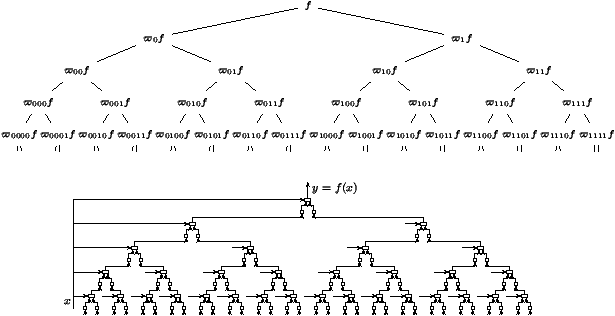Figure 4: The infinite synchronous decision tree (top) and its circuit realization (bottom).

Next, in order to be computable by some physical device, a digital function must be causal, but also representable by some finite system. To formalize this, we introduce the notion of on-line computable function: by this is meant a function such that the collection of all predictors of all orders forms a finite set. In this case, the (infinite) tree can be converted to a (finite) graph5 by identifying nodes of the SDT associated with functions that are equal. The resulting graph is called the synchronous decision diagram (SDD) and it is obtained by a simple algorithm: (i) build the infinite SDT for f; (ii) systematically share all the subexpressions generated during this process. (Optionally, one may also consider functions fg to be isomorphic if either f=g or fg; in that case the SDD will also involve logical negation gates but will be more compact.)

When presented as above, the SDD algorithm looks like an infinite process. However, it can be seen  that if a function is realizable by a finite transducer (i.e., an automaton with output), then the SDD algorithm terminates in finite time. In fact, the SDD algorithm provides an integrated alternative to the classical design of sequential circuits.6

In order to illustrate the SDD concept, we apply it now to the design of a circuit that takes as input a stream of bits (xt) meant to represent the real number x=åt³0xt2-t and produces as output the stream (yt) where the real number h=åt³0yt2-t satisfies h=(1/3) x. Introduce the integers
x(t)=2t
 å s£ t
xs
2s
,   y(t)=2t
 å s£ t
ys
2s
,   t³ 0,
and the carry rt defined by
x(t)=3y(t)+rt,   0£ rt<3,   t³ 0.
An easy calculation that mimics high school arithmetics yields
2rt+xt+1=3yt+1+rt+1,   t³ 0.
These formulæ show that the function h=x/3 is causal and that the bit yt+1=ft+1(x0,...,xt+1) depends only on the last input bit together with the ``carry'' rt that is inherited from past history. The carry can only assume three values and accordingly the number of predictors is finite, to the effect that the SDT has only six nodes. Thus, f is on-line computable. Figure 5 shows the result of the construction. (In the diagram, a transition denoted by a/b is triggered by reading the bit a and results in producing the bit b.)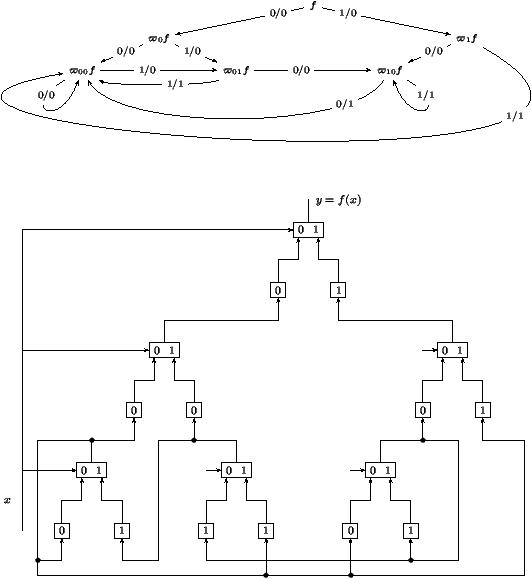Figure 5: The `(1/3) x' function: its abstract SDD representation (top) and the circuit realization (bottom).

## 4   Formal Power Series Representations of SDD's

A causal function f is characterized by its truth table. This is the power series representation Tf, an element of F2[[z]] defined as
T f(z):=
 å t³0
 å x0,...,xtÎ B
ft(x0,...,xt) z
 b(1x0... xt)-2
.
(The correction of -2 in the exponent is a convenience chosen to ensure that exponents start at 0.) We shall refer to Tf as the truth-table representation of f. This notion extends in a natural way the corresponding definition for combinational functions. Indeed, an alternative definition of Tf for causal functions is as follows: take Ft as the truth table of ft in ``listed'' form, and build the truth table of f in ``listed'' form by
 F0F1F2... = [ f0(0)f0(1) ] [ f1(00)f1(01)f1(10)f1(11) ] [ f2(000)f2(001)... f2(111) ] ... ;
then F(z)=Tf(z) satisfies a sort of a ``generating function relation,''
 F(z)= [ f0(0)+zf0(1) ] +z2 [ f1(00)+zf1(01)+z2f1(10)+z3f1(11) ]
 +z6 [ f2(000)+zf2(001)+··· +z7f2(111) ] +···,
so that there is a simple relation between truth tables of combinational functions and of sequential functions:
F(z)=
 å t³ 0
z
 2t+1-2
Tft(z).

Equipped with these definitions, we observe the action of sections,
 S0F(z)= [ f0(0) ] +z [ f1(00)+zf1(10) ] +z3 [ f2(000)+zf2(010)+··· +z3f2(110) ] +··· ,
 S1F(z)= [ f0(1) ] +z [ f1(01)+zf1(11) ] +z3 [ f2(001)+zf2(011)+··· +z3f2(111) ] +···,
which entails
S0F(z)=
 å t³ 0
z
 2t-1
S0Ft(z)= f0(0)+z
 å t³ 0
z
 2t+1-2
S0Ft+1(z),
S1F(z)=
 å t³ 0
z
 2t-1
S1Ft(z)= f0(1)+z
 å t³ 0
z
 2t+1-2
S1Ft+1(z).
This provides a direct relation between the sections of the truth table of any causal f and the predictors of f, namely,
S0(Tf)(z)=f0(0)+zT(v0f)(z),     S1(Tf)(z)=f0(1)+zT(v1f)(z).
Now, by definition, f is on-line computable when its predictors lie in a finite set. The equation above shows that this is equivalent to the finiteness of vector space over F2(z) of all the (iterated) sections of the truth table. The connection is thereby established with what is otherwise known as automatic series;7 see the foundational paper by Christol et al. , Dumas's thesis , and several summaries in previous issues of the Algorithms Seminar Proceedings. We state:

Theorem 1   The truth table Tf of an online computable function f is a 2-automatic series. Consequently, it is an algebraic function over the field F2(z).
Each causal finite function f may thus be represented by a bivariate characteristic polynomial P(z,y) so that the truth table Tf is the only root yÎF2[[z]] of P(z,y)=0. Conceivably, this theorem opens an avenue to circuit design and verification by means of polynomial elimination algorithms---typically, Gröbner bases. Given the superexponential complexity of algebraic elimination, it seems however to the authors of the summary that a direct approach based on linear algebra (in accordance with standard techniques of 2-automatic series ) should yield decision procedures of lower complexity.

## 5   From Circuits to Functions

In this section, we show how to put to good use the formalism introduced above in order to analyse circuits: starting from a given circuits, the goal is to determine a mathematical specification of what it does. Note that the dual problem of synthesis has been already implicitly tackled on the occasion of the ``one-third'' function (x|®x/3).

Let us first consider a circuit that takes as input a stream of bits (gt) and produces the stream (ht) which is the same stream delayed by 1 in time. In other words, we have h0=z0 (the initialization value) and ht=gt-1 for t³1. In the context of a finite circuit, the values ht are described by their truth table and they depend on the global input sequence x=(xt)t³0 of the circuit. Thus, in terms of the finite boolean functions gt(x0,...,xt) and ht(x0,...,xt), we have
h0=z0,   ht(x0,...,xt)=gt-1(x0,...,xt-1)   for t³ 1.
This relation translates into a relation between the truth tables of the input (G) and the output (H) of the register,
 H(z)=(1+z) ( z0+z2G(z2) ) .     (1)
Thus, in the formal power series representation, a register operates by way of the ``Mahlerian operator,'' G(z)|® G(z2).

Consider next a multiplexer that takes as input two streams of bits a(x) and b(x) (themselves causal functions of the input stream x) and assume that control is achieved by the input stream x. The output m(x) is a causal function defined by
 mt(x0,...,xt)=mux ( xt,at(x0,...,xt),bt(x0,...,xt) ) ,
which we abbreviate as
 m(x)=mux ( x,a(x),b(x) ) .
A little reflection shows that the truth table of m is obtained by suitably merging the truth tables of a and b as follows
 A a0(0) a0(1) a1(00) a1(01) a1(10) a1(11) a2(000) a2(001) ... B b0(0) b0(1) b1(00) b1(01) b1(10) b1(11) b2(000) b2(001) ... M b0(0) a0(1) b1(00) a1(01) b1(10) a1(11) b2(000) a2(001) ...  .
This relation translates into
M(z)=(S0B)(z2)+z(S1A)(z2),     (2)
which now involves a blend of sectioning and Mahlerian operators.

Now, a finite circuit can be translated into a system of fixed-point equations: to each entity is associated its truth table; then relations (1) and (2) (used repeatedly) provide the system of equations. Here is an application to a circuit discussed in . This circuit comprises one inverter (represented by a circle), two multiplexers, and one register that is initially set at 0. The upper entry of the leftmost multiplexer receives a continuous stream of 1's which is represented by 1.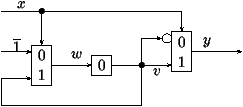What is required is to verify that the circuit computes the function
x |® h=x+1,
where the input and output streams are now interpreted as dyadic numbers, that is
x=
 å t³0
xt 2t,   h =
 å t³0
yt 2t,    x,y ÎZ2.
To each of the flows, y, v, w, one associates its truth table, respectively Y(z),V(z),W(z). Given the rules (1) and (2), the structural description of the circuit is translated (compiled!) into the system of equations:
Y(z)
= æ
ç
ç
è
S0 æ
ç
ç
è
1
1-z
+V ö
÷
÷
ø
ö
÷
÷
ø
(z2)+z ( S1V ) (z2) =
1
1-z2
+ ( S0V ) (z2)+z ( S1V ) (z2)

=
1
1-z2
+V(z),
V(z) =(1+z)z2W(z2),
W(z)
= æ
ç
ç
è
S0
1
1-z
ö
÷
÷
ø
(z2)+z ( S1V ) (z2) =
1
1-z2
+z ( S1V ) (z2).

In order to understand the function computed by the circuit, we proceed to solve this system. The second equation provides S1V(z)=zW(z), a relation that, when carried into the third equation, gives:
W(z)=
1
1-z2
+z3W(z2).
Such a functional equation is now easily solved by iteration,
W(z)=
 +¥ å k=0
z
 3(2k-1)
1-z
 2k+1
,
and this form entails in turn
Y(z)=
 +¥ å k=0
z
 3 · 2k-1
1-z
 2k+1
+
 +¥ å k=0
z
 3· 2k
1-z
 2k+1
 = [ 1 ] + z2 [ z+z2 ] + z6 [ z+z3+z5+z6 ] + z14 [ z+··· ] +··· .
From there, it is an easy exercise (left to the reader) to check that the truth table Y(z) is equal to the truth table corresponding to the dyadic function x|® x+1.

## 6   Conclusion

Due to constraints already evoked, we could only scratch the surface in this brief8 seminar summary. The point of view developed in the talk bases itself further on the existence of isomorphisms between various domains. For instance, as we have seen, the boolean domain may be viewed as B or F2; boolean functions are representable as elements of F2[z]; on-line computable functions are equivalent to algebraic elements of F2[[z]] and to 2-automatic series. There exist several other interesting connections, for instance, with the ring of dyadic integers Z2 that form one of the conceptual basis of the original paper . Such isomorphisms do increase the expressive power of the SDD formalism that we have opted to develop here only over  B while making use of representations in F2[[z]].

There is also great practical potential in the algorithms associated with the SDD concept. Quoting from Vuillemin: The SDD of f is a cyclic data structure, which represents the minimal finite state machine for f. In the worst case, its size is doubly exponential in the size of f. However, efficient algorithms exist to operate on the SDD representations with the following characteristics: constant time9 for f(l x), f(1+l x); linear time for ¬ f(x), l f(x), the inverse g(f(x))=x, and the fixed point y =l g(x,y); quadratic time for the composition f(g(x)) and for boolean operations, f(x) Ù f(y), etc; cubic time for the more general composition f(g(x),h(x)). The SDD opens an approach to sequential circuit synthesis and verification whose implementation is straightforward in a high-level language, and which can cope automatically with synchronous circuits of limited size.

## References


Bryant (Randal E.). -- Graph-based algorithms for boolean function manipulation. IEEE Transactions on Computers, vol. C-35, n°8, 1986, pp. 679--691.


Bryant (Randal E.). -- Symbolic boolean manipulation with ordered binary decision diagrams. ACM Computing Surveys, vol. 24, n°3, 1992, pp. 293--318.


Christol (G.), Kamae (T.), Mendès France (M.), and Rauzy (G.). -- Suites algébriques, automates et substitutions. Bulletin de la Société Mathématique de France, vol. 108, n°4, 1980, pp. 401--419.


Dumas (Philippe). -- Récurrences mahlériennes, suites automatiques, études asymptotiques. -- Institut National de Recherche en Informatique et en Automatique, Rocquencourt, 1993, 241p. Thèse, Université de Bordeaux I, Talence, 1993.


Flajolet (Philippe), Sipala (Paolo), and Steyaert (Jean-Marc). -- Analytic variations on the common subexpression problem. In Paterson (M. S.) (editor), Automata, languages and programming. Lecture Notes in Computer Science, vol. 443, pp. 220--234. -- Springer, New York, 1990. Proceedings of the 17th ICALP Conference, Warwick, July 1990.


Liaw (Heh Tyan) and Lin (Chen Shang). -- On the OBDD-representation of general boolean functions. IEEE Transactions on Computers, vol. 41, n°6, 1992, pp. 661--664.


van Leeuwen (Jan) (editor). -- Handbook of theoretical computer science. Vol. A. -- Elsevier Science Publishers, Amsterdam, 1990, x+996p. Algorithms and complexity.


Vuillemin (Jean E.). -- On circuits and numbers. IEEE Transactions on Computers, vol. 43, n°8, 1994, pp. 868--879.

1
Editor's Note. I acknowledge the promptitude of the authors of the summary. Especially their promptitude to renegociate deadlines.
2
The BDD's described here are sometimes called OBDD's, where the `O' stands for ``ordered'' and refers to a fixed ordering on boolean variables.
3
In the worst case, a BDD contains up to O(2n/n) nodes. A similar bound  even holds on average, for a random boolean function. Such properties are also related to a famous theorem of Shannon and Muller [7, p. 763] to the effect that almost all boolean functions have minimal circuit complexity of the order of 2n/n. This theoretical discussion is however to be counterbalanced by the fact that functions destined to be realized in silicon are seldom chosen at random!
4
The structure and size of a BDD depends on the ordering of variables. Several heuristics have been developed in order to try and come up with ``good'' orders.
5
Observe that, as opposed to the case of combinational circuits, the corresponding graph is no longer acyclic since nodes at different levels in the tree may be collapsed.
6
A classical construction starts from a specification of a finite automaton and stores the current state of the automaton in binary registers while realizing the transition function by means of a combinational circuit (itself possibly optimized via BDD's).
7
A sequence is defined to be automatic if its nth element is produced by a finite transducer applied to the binary representation of n; a series is automatic if its sequence of coefficients is automatic. Equivalent characterizations of automatic series are as algebraic elements over F2(z) or as solutions to Mahlerian equations; refer to [3, 4].
8
Editor's Note. I wish to express my true gratitude to the authors of the summary for not exceeding three times the expected length of a typical summary.
9
Dyadic interpretations (Z2) are understood in the first two examples.

This document was translated from LATEX by HEVEA.